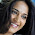## Monday, February 22, 2016

### Conversion between String, NSString, NSData and [UInt8] array in Swift

Update - May 19, 2017 Swift 3.1 (Xcode 8.3.2)

The code below shows how to convert a string to a UInt8 array and then back to string. The code includes:

1. Step-by-step conversion between the String, NSString, NSData, and [UInt8] in this procedure:

String -> NSString -> NSData -> [UInt8] -> NSData -> NSString -> String

2. Direct conversion between String and [UInt8]:

String -> [UInt8] -> String

Swift Code:

Update - May 19, 2017 Swift 3.1 (Xcode 8.3.2)

let myString = "This is my string."

//1.Conversion between the String, NSString, NSData, and [UInt8]
//Convert String to NSString
let myNSString = myString as NSString

//Convert NSString to NSData
let myNSData = myNSString.data(using: String.Encoding.utf8.rawValue)!

//Convert NSData to [UInt8] array
let myArray = [UInt8](myNSData)

//Convert [UInt8] to NSData
let resultNSData = NSData(bytes: myArray, length: myArray.count)

//Convert NSData to NSString
let resultNSString = NSString(data: resultNSData as Data, encoding: String.Encoding.utf8.rawValue)!

//Convert NSString to String

let resultString = resultNSString as String

print(resultString)

//2.Direct conversion between the String and [UInt8]
//Directly convert string to [UInt8]
let directArray : [UInt8] = Array(myString.utf8)

//Directly convert [UInt8] to String
let directResultString = NSString(bytes: directArray, length:
directArray.count, encoding: String.Encoding.utf8.rawValue)! as String
print(directResultString)

Original Post: Feb. 22, 2016 with Swift 2.1

let myString = "This is my string."

//1.Conversion between the String, NSString, NSData, and [UInt8]
//Convert String to NSString
let myNSString = myString as NSString

//Convert NSString to NSData
let myNSData = myNSString.dataUsingEncoding(NSUTF8StringEncoding)!

//Get length of [UInt8]
let length = myNSData.length

//Convert NSData to [UInt8] array
var myArray = [UInt8](count: length, repeatedValue: 0)
myNSData.getBytes(&myArray, length: length)

//Convert [UInt8] to NSData
let resultNSData = NSData(bytes: &myArray, length: length)

//Convert NSData to NSString
let resultNSString = NSString(data: resultNSData, encoding: NSUTF8StringEncoding)!

//Convert NSString to String

let resultString = resultNSString as String

print(resultString)

//2.Direct conversion between the String and [UInt8]
//Directly convert string to [UInt8]
let directArray : [UInt8] = Array(myString.utf8)

//Directly convert [UInt8] to String
let directResultString = NSString(bytes: directArray, length:
directArray.count, encoding: NSUTF8StringEncoding) as! String

1.2.3.